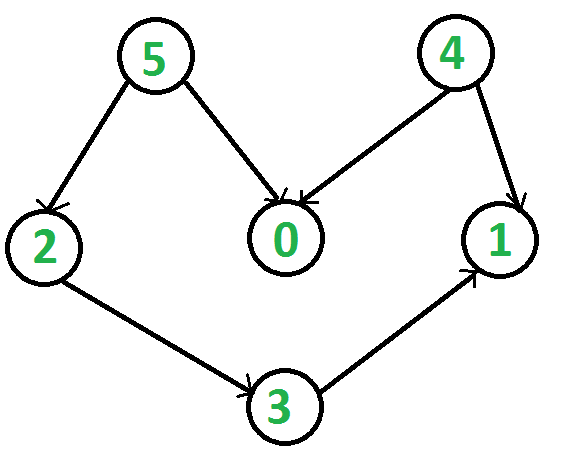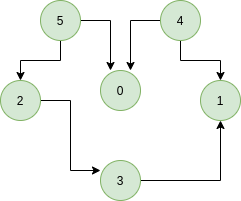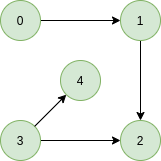Open In App
Related Articles

# Kahn’s algorithm for Topological Sorting

Topological sorting for Directed Acyclic Graph (DAG) is a linear ordering of vertices such that for every directed edge uv, vertex u comes before v in the ordering. Topological Sorting for a graph is not possible if the graph is not a DAG.
For example, a topological sorting of the following graph is “5 4 2 3 1 0?. There can be more than one topological sorting for a graph. For example, another topological sorting of the following graph is “4 5 2 0 3 1″. The first vertex in topological sorting is always a vertex with an in-degree of 0 (a vertex with no incoming edges).Let’s look at a few examples with proper explanation,
Example:

Input:Output: 5 4 2 3 1 0
Explanation: The topological sorting of a DAG is done in a order such that for every directed edge uv, vertex u comes before v in the ordering. 5 has no incoming edge. 4 has no incoming edge, 2 and 0 have incoming edge from 4 and 5 and 1 is placed at last.
Input:Output: 0 3 4 1 2
Explanation: 0 and 3 have no incoming edge, 4 and 1 has incoming edge from 0 and 3. 2 is placed at last.

A DFS based solution to find a topological sort has already been discussed.
Solution: In this article, we will see another way to find the linear ordering of vertices in a directed acyclic graph (DAG). The approach is based on the below fact:
A DAG G has at least one vertex with in-degree 0 and one vertex with out-degree 0
Proof: There’s a simple proof to the above fact that a DAG does not contain a cycle which means that all paths will be of finite length. Now let S be the longest path from u(source) to v(destination). Since S is the longest path there can be no incoming edge to u and no outgoing edge from v, if this situation had occurred then S would not have been the longest path
=> indegree(u) = 0 and outdegree(v) = 0

Intuition:

Topological sorting is a kind of dependencies problem so, we can start with the tasks which do not have any dependencies and can be done straight away or simply if we talk about in the term of node,

• We will always try to execute those nodes that have outdegree 0.
• Then after execution of all those 0 outdegrees, we will execute which are directly dependent on currently resolved tasks (currently resolved tasks’ outdegrees will become 0 now) and so on will execute all other tasks.

We look closely we are doing these executions are done level-wise or in a Breadth-first search (BFS) manner. Similarly, we can also perform the same task for indegree=0.

Algorithm: Steps involved in finding the topological ordering of a DAG:
Step-1: Compute in-degree (number of incoming edges) for each of the vertex present in the DAG and initialize the count of visited nodes as 0.
Step-2: Pick all the vertices with in-degree as 0 and add them into a queue (Enqueue operation)
Step-3: Remove a vertex from the queue (Dequeue operation) and then.

1. Increment the count of visited nodes by 1.
2. Decrease in-degree by 1 for all its neighbouring nodes.
3. If the in-degree of neighbouring nodes is reduced to zero, then add it to the queue.

Step 4: Repeat Step 3 until the queue is empty.
Step 5: If the count of visited nodes is not equal to the number of nodes in the graph then the topological sort is not possible for the given graph.
How to find the in-degree of each node?
There are 2 ways to calculate in-degree of every vertex:
1. Take an in-degree array which will keep track of
Traverse the array of edges and simply increase the counter of the destination node by 1.

```for each node in Nodes
indegree[node] = 0;
for each edge(src, dest) in Edges
indegree[dest]++```

Time Complexity: O(V+E)

2. Traverse the list for every node and then increment the in-degree of all the nodes connected to it by 1.

```    for each node in Nodes
If (list[node].size()!=0) then
for each dest in list
indegree[dest]++;```

Time Complexity: The outer for loop will be executed V number of times and the inner for loop will be executed E number of times, Thus overall time complexity is O(V+E).
The overall time complexity of the algorithm is O(V+E)

Below is the implementation of the above algorithm. The implementation uses method 2 discussed above for finding in degrees.

## C++

 `// A C++ program to print topological``// sorting of a graph using indegrees.``#include ``using` `namespace` `std;` `// Class to represent a graph``class` `Graph {``    ``// No. of vertices'``    ``int` `V;` `    ``// Pointer to an array containing``    ``// adjacency listsList``    ``list<``int``>* adj;` `public``:``    ``// Constructor``    ``Graph(``int` `V);` `    ``// Function to add an edge to graph``    ``void` `addEdge(``int` `u, ``int` `v);` `    ``// prints a Topological Sort of``    ``// the complete graph``    ``void` `topologicalSort();``};` `Graph::Graph(``int` `V)``{``    ``this``->V = V;``    ``adj = ``new` `list<``int``>[V];``}` `void` `Graph::addEdge(``int` `u, ``int` `v)``{``    ``adj[u].push_back(v);``}` `// The function to do``// Topological Sort.``void` `Graph::topologicalSort()``{``    ``// Create a vector to store``    ``// indegrees of all``    ``// vertices. Initialize all``    ``// indegrees as 0.``    ``vector<``int``> in_degree(V, 0);` `    ``// Traverse adjacency lists``    ``// to fill indegrees of``    ``// vertices.  This step``    ``// takes O(V+E) time``    ``for` `(``int` `u = 0; u < V; u++) {``        ``list<``int``>::iterator itr;``        ``for` `(itr = adj[u].begin();``             ``itr != adj[u].end(); itr++)``            ``in_degree[*itr]++;``    ``}` `    ``// Create an queue and enqueue``    ``// all vertices with indegree 0``    ``queue<``int``> q;``    ``for` `(``int` `i = 0; i < V; i++)``        ``if` `(in_degree[i] == 0)``            ``q.push(i);` `    ``// Initialize count of visited vertices``    ``int` `cnt = 0;` `    ``// Create a vector to store``    ``// result (A topological``    ``// ordering of the vertices)``    ``vector<``int``> top_order;` `    ``// One by one dequeue vertices``    ``// from queue and enqueue``    ``// adjacents if indegree of``    ``// adjacent becomes 0``    ``while` `(!q.empty()) {``        ``// Extract front of queue``        ``// (or perform dequeue)``        ``// and add it to topological order``        ``int` `u = q.front();``        ``q.pop();``        ``top_order.push_back(u);` `        ``// Iterate through all its``        ``// neighbouring nodes``        ``// of dequeued node u and``        ``// decrease their in-degree``        ``// by 1``        ``list<``int``>::iterator itr;``        ``for` `(itr = adj[u].begin();``             ``itr != adj[u].end(); itr++)` `            ``// If in-degree becomes zero,``            ``// add it to queue``            ``if` `(--in_degree[*itr] == 0)``                ``q.push(*itr);` `        ``cnt++;``    ``}` `    ``// Check if there was a cycle``    ``if` `(cnt != V) {``        ``cout << ``"There exists a cycle in the graph\n"``;``        ``return``;``    ``}` `    ``// Print topological order``    ``for` `(``int` `i = 0; i < top_order.size(); i++)``        ``cout << top_order[i] << ``" "``;``    ``cout << endl;``}` `// Driver program to test above functions``int` `main()``{``    ``// Create a graph given in the``    ``// above diagram``    ``Graph g(6);``    ``g.addEdge(5, 2);``    ``g.addEdge(5, 0);``    ``g.addEdge(4, 0);``    ``g.addEdge(4, 1);``    ``g.addEdge(2, 3);``    ``g.addEdge(3, 1);` `    ``cout << ``"Following is a Topological Sort of\n"``;``    ``g.topologicalSort();` `    ``return` `0;``}`

## Java

 `// A Java program to print topological``// sorting of a graph using indegrees``import` `java.util.*;` `// Class to represent a graph``class` `Graph {``    ``// No. of vertices``    ``int` `V;` `    ``// An Array of List which contains``    ``// references to the Adjacency List of``    ``// each vertex``    ``List adj[];``    ``// Constructor``    ``public` `Graph(``int` `V)``    ``{``        ``this``.V = V;``        ``adj = ``new` `ArrayList[V];``        ``for` `(``int` `i = ``0``; i < V; i++)``            ``adj[i] = ``new` `ArrayList();``    ``}` `    ``// Function to add an edge to graph``    ``public` `void` `addEdge(``int` `u, ``int` `v)``    ``{``        ``adj[u].add(v);``    ``}``    ``// prints a Topological Sort of the``    ``// complete graph``    ``public` `void` `topologicalSort()``    ``{``        ``// Create a array to store``        ``// indegrees of all``        ``// vertices. Initialize all``        ``// indegrees as 0.``        ``int` `indegree[] = ``new` `int``[V];` `        ``// Traverse adjacency lists``        ``// to fill indegrees of``        ``// vertices. This step takes``        ``// O(V+E) time``        ``for` `(``int` `i = ``0``; i < V; i++) {``            ``ArrayList temp``                ``= (ArrayList)adj[i];``            ``for` `(``int` `node : temp) {``                ``indegree[node]++;``            ``}``        ``}` `        ``// Create a queue and enqueue``        ``// all vertices with indegree 0``        ``Queue q``            ``= ``new` `LinkedList();``        ``for` `(``int` `i = ``0``; i < V; i++) {``            ``if` `(indegree[i] == ``0``)``                ``q.add(i);``        ``}` `        ``// Initialize count of visited vertices``        ``int` `cnt = ``0``;` `        ``// Create a vector to store result``        ``// (A topological ordering of the vertices)``        ``Vector topOrder = ``new` `Vector();``        ``while` `(!q.isEmpty()) {``            ``// Extract front of queue``            ``// (or perform dequeue)``            ``// and add it to topological order``            ``int` `u = q.poll();``            ``topOrder.add(u);` `            ``// Iterate through all its``            ``// neighbouring nodes``            ``// of dequeued node u and``            ``// decrease their in-degree``            ``// by 1``            ``for` `(``int` `node : adj[u]) {``                ``// If in-degree becomes zero,``                ``// add it to queue``                ``if` `(--indegree[node] == ``0``)``                    ``q.add(node);``            ``}``            ``cnt++;``        ``}` `        ``// Check if there was a cycle``        ``if` `(cnt != V) {``            ``System.out.println(``                ``"There exists a cycle in the graph"``);``            ``return``;``        ``}` `        ``// Print topological order``        ``for` `(``int` `i : topOrder) {``            ``System.out.print(i + ``" "``);``        ``}``    ``}``}``// Driver program to test above functions``class` `Main {``    ``public` `static` `void` `main(String args[])``    ``{``        ``// Create a graph given in the above diagram``        ``Graph g = ``new` `Graph(``6``);``        ``g.addEdge(``5``, ``2``);``        ``g.addEdge(``5``, ``0``);``        ``g.addEdge(``4``, ``0``);``        ``g.addEdge(``4``, ``1``);``        ``g.addEdge(``2``, ``3``);``        ``g.addEdge(``3``, ``1``);``        ``System.out.println(``            ``"Following is a Topological Sort"``);``        ``g.topologicalSort();``    ``}``}`

## Python3

 `# A Python program to print topological sorting of a graph``# using indegrees``from` `collections ``import` `defaultdict` `# Class to represent a graph``class` `Graph:``    ``def` `__init__(``self``, vertices):``        ``self``.graph ``=` `defaultdict(``list``) ``# dictionary containing adjacency List``        ``self``.V ``=` `vertices ``# No. of vertices` `    ``# function to add an edge to graph``    ``def` `addEdge(``self``, u, v):``        ``self``.graph[u].append(v)`  `    ``# The function to do Topological Sort.``    ``def` `topologicalSort(``self``):``        ` `        ``# Create a vector to store indegrees of all``        ``# vertices. Initialize all indegrees as 0.``        ``in_degree ``=` `[``0``]``*``(``self``.V)``        ` `        ``# Traverse adjacency lists to fill indegrees of``           ``# vertices.  This step takes O(V + E) time``        ``for` `i ``in` `self``.graph:``            ``for` `j ``in` `self``.graph[i]:``                ``in_degree[j] ``+``=` `1` `        ``# Create an queue and enqueue all vertices with``        ``# indegree 0``        ``queue ``=` `[]``        ``for` `i ``in` `range``(``self``.V):``            ``if` `in_degree[i] ``=``=` `0``:``                ``queue.append(i)` `        ``# Initialize count of visited vertices``        ``cnt ``=` `0` `        ``# Create a vector to store result (A topological``        ``# ordering of the vertices)``        ``top_order ``=` `[]` `        ``# One by one dequeue vertices from queue and enqueue``        ``# adjacents if indegree of adjacent becomes 0``        ``while` `queue:` `            ``# Extract front of queue (or perform dequeue)``            ``# and add it to topological order``            ``u ``=` `queue.pop(``0``)``            ``top_order.append(u)` `            ``# Iterate through all neighbouring nodes``            ``# of dequeued node u and decrease their in-degree``            ``# by 1``            ``for` `i ``in` `self``.graph[u]:``                ``in_degree[i] ``-``=` `1``                ``# If in-degree becomes zero, add it to queue``                ``if` `in_degree[i] ``=``=` `0``:``                    ``queue.append(i)` `            ``cnt ``+``=` `1` `        ``# Check if there was a cycle``        ``if` `cnt !``=` `self``.V:``            ``print` `(``"There exists a cycle in the graph"``)``        ``else` `:``            ``# Print topological order``            ``print` `(top_order)`  `g ``=` `Graph(``6``)``g.addEdge(``5``, ``2``);``g.addEdge(``5``, ``0``);``g.addEdge(``4``, ``0``);``g.addEdge(``4``, ``1``);``g.addEdge(``2``, ``3``);``g.addEdge(``3``, ``1``);` `print` `(``"Following is a Topological Sort of the given graph"``)``g.topologicalSort()` `# This code is contributed by Neelam Yadav`

## C#

 `// C# program to print topological``// sorting of a graph using indegrees.` `using` `System;``using` `System.Collections.Generic;` `// Class to represent a graph``public` `class` `Graph {``  ``// No. of vertices'``  ``private` `int` `V;` `  ``// Pointer to an array containing``  ``// adjacency listsList``  ``private` `List<``int``>[] adj;` `  ``// Constructor``  ``public` `Graph(``int` `V)``  ``{``    ``this``.V = V;``    ``adj = ``new` `List<``int``>[ V ];``    ``for` `(``int` `i = 0; i < V; i++)``      ``adj[i] = ``new` `List<``int``>();``  ``}` `  ``// Function to Add an edge to graph``  ``public` `void` `AddEdge(``int` `u, ``int` `v) { adj[u].Add(v); }` `  ``// The function to do Topological Sort.``  ``public` `void` `TopologicalSort()``  ``{``    ` `    ``// Create a list to store``    ``// indegrees of all``    ``// vertices. Initialize all``    ``// indegrees as 0.``    ``int``[] in_degree = ``new` `int``[V];` `    ``// Traverse adjacency lists``    ``// to fill indegrees of``    ``// vertices. This step``    ``// takes O(V+E) time``    ``for` `(``int` `u = 0; u < V; u++) {``      ``foreach``(``int` `itr ``in` `adj[u]) in_degree[itr]++;``    ``}` `    ``// Create an queue and enqueue``    ``// all vertices with indegree 0``    ``Queue<``int``> q = ``new` `Queue<``int``>();``    ``for` `(``int` `i = 0; i < V; i++)``      ``if` `(in_degree[i] == 0)``        ``q.Enqueue(i);` `    ``// Initialize count of visited vertices``    ``int` `cnt = 0;` `    ``// Create a vector to store``    ``// result (A topological``    ``// ordering of the vertices)``    ``List<``int``> top_order = ``new` `List<``int``>();` `    ``// One by one dequeue vertices``    ``// from queue and enqueue``    ``// adjacents if indegree of``    ``// adjacent becomes 0``    ``while` `(q.Count > 0)``    ``{``      ` `      ``// Extract front of queue``      ``// (or perform dequeue)``      ``// and Add it to topological order``      ``int` `u = q.Dequeue();``      ``top_order.Add(u);` `      ``// Iterate through all its``      ``// neighbouring nodes``      ``// of dequeued node u and``      ``// decrease their in-degree``      ``// by 1``      ``foreach``(``int` `itr ``in` `adj[u])``      ``{` `        ``// If in-degree becomes zero,``        ``// Add it to queue``        ``if` `(--in_degree[itr] == 0)``          ``q.Enqueue(itr);``      ``}` `      ``cnt++;``    ``}` `    ``// Check if there was a cycle``    ``if` `(cnt != V) {``      ``Console.WriteLine(``        ``"There exists a cycle in the graph"``);``      ``return``;``    ``}` `    ``// Print topological order``    ``for` `(``int` `i = 0; i < top_order.Count; i++)``      ``Console.Write(top_order[i] + ``" "``);``    ``Console.WriteLine();``  ``}``}` `// Driver program to test above functions``public` `class` `GFG {``  ``static` `void` `Main(``string``[] args)` `  ``{` `    ``// Create a graph given in the``    ``// above diagram``    ``Graph g = ``new` `Graph(6);``    ``g.AddEdge(5, 2);``    ``g.AddEdge(5, 0);``    ``g.AddEdge(4, 0);``    ``g.AddEdge(4, 1);``    ``g.AddEdge(2, 3);``    ``g.AddEdge(3, 1);` `    ``Console.WriteLine(``      ``"Following is a Topological Sort of"``);``    ``g.TopologicalSort();``  ``}``}` `// This code is contributed by cavi4762.`

## Javascript

 ``

Output

```Following is a Topological Sort of
4 5 2 0 3 1 ```

Complexity Analysis:

• Time Complexity: O(V+E).
The outer for loop will be executed V number of times and the inner for loop will be executed E number of times.
• Auxiliary Space: O(V).
The queue needs to store all the vertices of the graph. So the space required is O(V)

Application of Kahn’s algorithm for Topological Sort:

1.Course sequencing: Courses at universities frequently have prerequisites for other courses. The courses can be scheduled using Kahn’s algorithm so that the prerequisites are taken before the courses that call for them.

2.Management of software dependencies: When developing software, libraries and modules frequently rely on other libraries and modules. The dependencies can be installed in the proper order by using Kahn’s approach.

3.Scheduling tasks: In project management, activities frequently depend on one another. The tasks can be scheduled using Kahn’s method so that the dependent tasks are finished before the tasks that depend on them.

4.Data processing: In data processing pipelines, the outcomes of some processes may be dependent. The stages can be carried out in the right order by using Kahn’s algorithm.

5.Circuit design: In the creation of an electronic circuit, some components may be dependent on the output of others. The components can be joined in the right order by using Kahn’s technique.